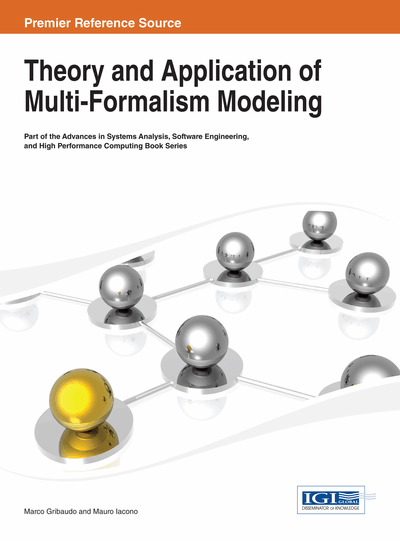# A Petri Net-Based Tool for the Analysis of Generalized Continuous Time Bayesian Networks

Daniele Codetta-Raiteri (University of Piemonte Orientale, Italy) and Luigi Portinale (University of Piemonte Orientale, Italy)
DOI: 10.4018/978-1-4666-4659-9.ch007
Available
\$37.50
No Current Special Offers

## Abstract

A software tool for the analysis of Generalized Continuous Time Bayesian Networks (GCTBN) is presented. GCTGBN extend CTBN introducing in addition to continuous time-delayed variables, non-delayed or “immediate” variables. The tool is based on the conversion of a GCTBN model into a Generalized Stochastic Petri Net (GSPN), which is an actual mean to perform the inference (analysis) of the GCTBN. Both the inference tasks (prediction and smoothing) can be performed in this way. The architecture and the methodologies of the tool are presented. In particular, the conversion rules from GCTBN to GSPN are described, and the inference algorithms exploiting GSPN transient analysis are presented. A running example supports their description: a case study is modelled as a GCTBN and analyzed by means of the tool. The results are verified by modelling and analyzing the system as a Dynamic Bayesian Network, another form of Bayesian Network, assuming discrete time.
Chapter Preview
Top

## Introduction

We present a software tool for the analysis of Generalized Continuous Time Bayesian Network (GCTBN) (Codetta & Portinale, 2010). The tool is based on the model-to-model transformation of a GCTBN into a Generalized Stochastic Petri Net (GSPN) (Ajmone et al., 1995).

GCTBN are a particular form of Bayesian Network (BN) (Langseth & Portinale, 2007), are characterized by a continuous time dimension, and contain two kinds of variables: delayed variables having a temporal evolution, and immediate variables whose value immediately changes according to the values of the parent variables.

The possibilities offered by this generalization, can be exploited in several applications. For example, in system reliability analysis, it is very practical to distinguish between system components (having a temporal evolution) and specific modules or subsystems, whose behavior has to be modeled for the analysis. This is shown by the case study presented in the following sections. Another case concerns Fault Tree Analysis (FTA) (Sahner et al., 1996) where basic events represent the system components with their failure rates, while non-basic events are logical gates identifying modules of the system under examination. In Dynamic Fault Trees (Bechta Dugan et al., 1992), logical gates identifying sub-modules, can be combined with dynamic gates, modeling time-dependent dependencies (usually assuming continuous time) among components or sub-modules. Also in this case, it is very important to distinguish, at the modeling level, between delayed and immediate entities (see also Portinale et al., 2007). Of course, similar considerations apply in other tasks as well, as in medical diagnosis, financial forecasting, biological process modeling, etc.

GCTBN and GSPN share the same stochastic process composed by tangible states characterized by an actual duration, and vanishing states where the system spend no time. This is the reason why a GCTBN can converted into GSPN. The tool exploits GSPN transient analysis to perform the inference of the GCTBN. This means computing the probability distribution of the queried variables at a specific time, conditioned by the observation of the values of other variables at particular times. However, a single transient analysis of the equivalent GSPN is not enough to perform the inference (analysis) of a GCTBN. The inference must take into account the observations (evidences) and their times of occurrence. So, the GSPN model has to be modified and analyzed at each time of interest, in order to represent and consider the observations. The results obtained at each time, must be properly combined in order to return the effective probability distribution of the queried variables.

Solution techniques for GSPN have received a lot of attention, especially with respect to the possibility of solving the underlying state space efficiently (Miner, 2007). For this reason, we exploit GSPN analysis for GCTBN inference. At the moment, this is the only method developed to solve GCTBN models. This method will be useful in the future to verify inference results if a direct solver will be developed for GCTBN. CTBN solution methods (Nodelman et al., 2005) may be extended to deal with GCTBN. However, CTBN solution usually provides approximate results, while the GSPN based approach described in this paper, returns exact results because it exploits GSPN transient analysis.

The presentation of the tool is supported by a running example, and we describe the formalisms involved in the tool (GCTBN and GSPN) in terms of modelling primitives and analysis tasks. In particular, we present the conversion rules from GCTBN to GSPN, and the inference algorithms exploiting GSPN transient analysis. The results obtained for the running example are verified by modelling and analyzing the system as a Dynamic Bayesian Network (DBN) (Murphy, 2002).

## Complete Chapter List

Search this Book:
Reset